# Prime number

Jan wrote any number from 1 to 20. What is the probability that he wrote the prime number?

p =  0.4

### Step-by-step explanation: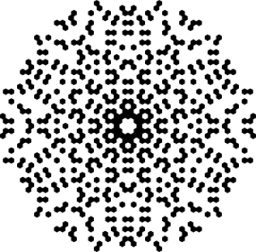Did you find an error or inaccuracy? Feel free to write us. Thank you!Tips to related online calculators
Would you like to compute count of combinations?

## Related math problems and questions:

• Raffle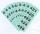In the pool is numbers from 1 to 115. What is the probability that a randomly selected number is not a prime number?
• 9.A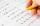9.A to attend more than 20 students but fewer than 40 students. A third of the pupils wrote a math test to mark 1, the sixth to mark 2, the ninth to mark 3. No one gets mark 4. How many students of class 9.A wrote a test to mark 5?
• Balls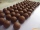From the bag with numbered balls (numbers 1,2,3,. ..20) we pick one ball. What is the probability of choosing a number containing 1?
• Drawing from a hatWhen drawing numbers from a hat from 1 to 35, we select random given numbers. What is the probability that the drawn numbers will be divisible by 8 and 2?
• Air draftThe numbers 1,2,3,4,5 are written on five tickets on the table. Air draft randomly shuffled the tickets and composed a 5-digit number from them. What is the probability that he passed: and, the largest possible number b, the smallest possible number c, a
• ProbabilityHow probable is a randomly selected three-digit number divisible by five or seven?
• Three dice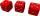What is the probability that when we roll three dice, we roll the numbers 1,2,3?
• Probability - tickets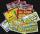What is the probability when you have 25 tickets in 5000 that you not wins the first (one) prize?
• DicesWe will throw two dice. What is the probability that the ratio between numbers on first and second dice will be 1:2?
• Event probability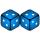The probability of event P in 8 independent experiments is 0.33. What is the probability that the event P occurs in one experiment (chance is the same)?
• RectangleIn a rectangle with sides, 8 and 9 mark the diagonal. What is the probability that a randomly selected point within the rectangle is closer to the diagonal than to any side of the rectangle?
• Five-digit numberAnna thinks of a five-digit number that is not divisible by three or four. If he increments each digit by one, it gets a five-digit number that is divisible by three. If he reduces each digit by one, he gets a five-digit number divisible by four. If it sw
• Z9-I-4Kate thought a five-digit integer. She wrote the sum of this number and its half at the first line to the workbook. On the second line wrote a total of this number and its one fifth. On the third row, she wrote a sum of this number and its one nines. Fina
• PediatricianPediatrician this month of 20 working days takes 8 days holidays. What is the probability that on Monday it will be at work?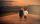Peter and Franta threw to the basket. Each had 20 attempts. Peter scored thirteen and twelve Franta. Give them percentage success.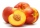There are 20 peaches in the pocket. 3 peaches are rotten. What is the probability that one of the randomly picked two peaches will be just one rotten?We roll two dice A. - what is the probability that the sum of the falling numbers is at most 4 B. - is at least 10 C. - is divisible by 5?